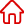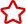﻿ php 將一個二維數組轉換成有父子關系的數組_關於PHP編程設為首頁 加入收藏

C語言|JAVA編程
Python編程

ASP編程|PHP編程
JSP編程

MYSQL數據庫|SqlServer數據庫
Oracle數據庫|DB2數據庫
程式師世界 >> 編程語言 >> 網頁編程 >> PHP編程 >> 關於PHP編程 >> php 將一個二維數組轉換成有父子關系的數組

php 將一個二維數組轉換成有父子關系的數組

<?
=
__construct(,  = ('id', 'pid'),  = 0->result = ->fields = ->root = ->
(->result  [[->fields]][] = ( ( = ();  > 0; -- (   =>  (!(, -> (!->->tmp = (, ->already[] =  (   =>  ([->fields] == ->tmp[0[][]['child'] = ->tmp[1->tmp = (, [->tmp = ->tmp =
recur_n(,  (   ([->fields] == ->arr[] =  ([->fields] != ->root) ->recur_n(, [->fields[1
recur_p( (  ->arr[] = [->fields[0 (['child']) ->recur_p(['child'
leaf( =  = ( == ) ? ->root :  ->tmp[
navi(->arr = ->recur_n(->result, (-> ->
leafid(->arr = ->arr[] = ->recur_p(->leaf( ->?>